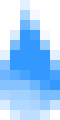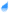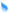# RAINDROPS - organic motion #2This is another experiment that attempts to simulate Organic Motion, much like "organic01." This one, however, tries to simulate raindrops rolling down a surface, like a window.

The whole thing is based on sine waves. Each "raindrop" is started at a random place on the "surface" to the left, and runs down it, with its horizontal position dictated by an equation for a sine wave. The equation is different for each raindrop. Also, for each raindrop, the equation is changed after it has run through one whole period (the period of the equation y = sin x is ).

I don't know how much sense that made.

This is very processor intensive, so your computer may get quite sluggish while looking at this. This is the reason that I am letting you decide how many raindrops to look at, to the left.

It looks very nice on Windows NT/2000, and might look decent on a Mac. If you happen to be using Windows 9x/ME, just imagine that the raindrops are all rolling smoothly down the surface to the left, instead of skipping slowly down it.

« back to Humor Tree